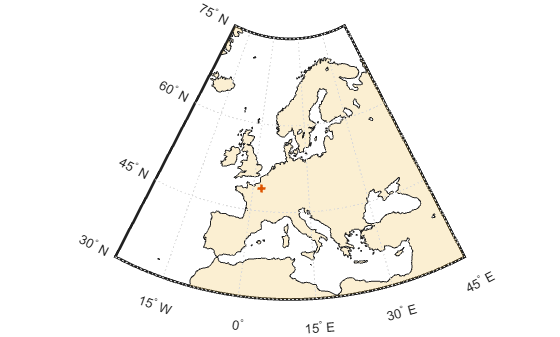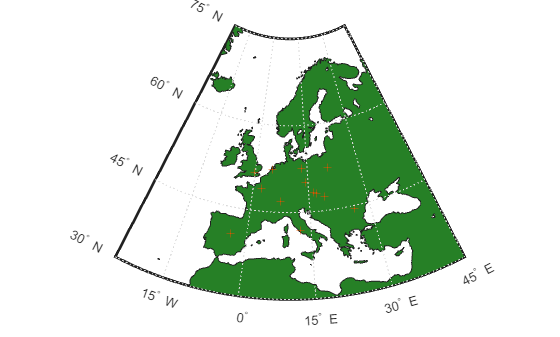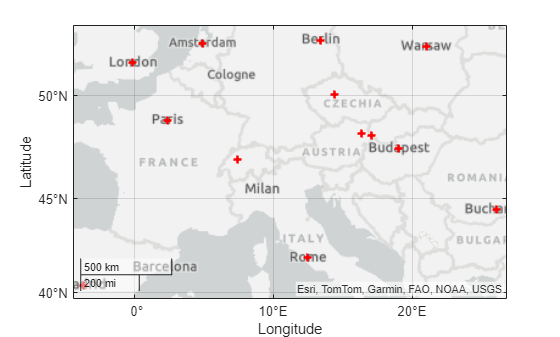geopoint

Geographic point vector

Description

A geopoint vector is a container object that holds geographic point coordinates and attributes. The points are coupled, such that the size of the latitude and longitude coordinate arrays are always equal and match the size of any dynamically added attribute arrays. Each entry of a coordinate pair and associated attributes, if any, represents a discrete element in the geopoint vector.

To create a geographic point shape for use with a geospatial table, create a geopointshape object instead.

Creation

Description

example

p = geopoint() constructs an empty geopoint vector with these default property settings:

p =

0x1 geopoint vector with properties:

Collection properties:
Geometry: 'point'
Feature properties:
Latitude: []
Longitude: []

example

p = geopoint(latitude,longitude) sets the Latitude and Longitude properties of geopoint vector p

example

p = geopoint(latitude,longitude,Name,Value) sets the Latitude and Longitude properties, then adds dynamic properties to the geopoint vector using Name,Value argument pairs. You can specify several name-value pair arguments in any order as Name1,Value1,...,NameN,ValueN.

example

p = geopoint(structArray) constructs a new geopoint vector from the fields of the structure, structArray.

• If structArray contains the field Lat, and does not contain a field Latitude, then the Lat values are assigned to the Latitude property. Similar behavior occurs when structArray contains the field Lon, and does not contain the field Longitude.

• If structArray contains both Lat and Latitude fields, then both field values are assigned to p. Similar behavior occurs for Lon and Longitude fields when both are present in structArray

• Other fields of structArray are assigned to p and become dynamic properties. Field values in structArray that are not numeric data types, string scalars, string arrays, character vectors, or cell arrays of numeric data types or character vectors are ignored.

example

p = geopoint(latitude,longitude,structArray) sets the Latitude and Longitude properties, and sets dynamic properties from the field values of the structure, structArray.

• If structArray contains the fields Lat, Latitude, Lon, or Longitude, then those field values are ignored.

Properties

expand all

Each element in a geopoint vector is considered a feature. For more about the property types in geopoint, see Collection Properties and Feature Properties.

Dynamic properties are new features that are added to a geopoint vector and that apply to each individual feature in the geopoint vector. You can attach new dynamic Feature properties to the object during construction with a Name,Value pair or after construction using dot (.) notation. This is similar to adding dynamic fields to a structure. For an example of adding dynamic Feature properties, see Construct Geopoint Vector Using Name-Value Pairs.

Type of geometry, specified as 'point'. For geopoint, Geometry is always 'point'.

Data Types: char | string

Latitude coordinates, specified as a numeric row or column vector.

Data Types: double | single

Longitude coordinates, specified as a numeric row or column vector.

Data Types: double | single

Information for the entire set of geopoint vector elements, specified as a scalar structure. You can add any data type to the structure.

• If Metadata is provided as a dynamic property Name in the constructor, and the corresponding Value is a scalar structure, then Value is copied to the Metadata property. Otherwise, an error is issued.

• If a Metadata field is provided by structArray, and both Metadata and structArray are scalar structures, then the Metadata field value is copied to the Metadata property value. If structArray is a scalar but the Metadata field is not a structure, then an error is issued. If structArray is not scalar, then the Metadata field is ignored.

Data Types: struct

Object Functions

 append Append features to geographic or planar vector cat Concatenate geographic or planar vector disp Display geographic or planar vector fieldnames Return dynamic property names of geographic or planar vector isempty Determine if geographic or planar vector is empty isfield Determine if dynamic property exists in geographic or planar vector isprop Determine if property exists in geographic or planar vector length Return number of elements in geographic or planar vector properties Return property names of geographic or planar vector rmfield Remove dynamic property from geographic or planar vector rmprop Remove property from geographic or planar vector size Return size of geographic or planar vector struct Convert geographic or planar vector to scalar structure vertcat Vertically concatenate geographic or planar vectors

Examples

collapse all

This example shows how to create a geopoint vector, specifying latitude and longitude coordinates, and display it.

Create Geopoint Containing Single Point and Display It

Create a geopoint vector using the latitude and longitude of Paris, France, and display it. When using a geopoint vector, the geometry of the constructed object is always 'point'.

lat = 48.8566;
lon = 2.3522;
p = geopoint(lat,lon)
p =
1×1 geopoint vector with properties:

Collection properties:
Geometry: 'point'
Feature properties:
Latitude: 48.8566
Longitude: 2.3522

Display the point in a plot. You can pass a geopoint vector directly to the geoshow command. geoshow can read the latitude and longitude values from the geopoint vector and also reads the geometry type.

geoshow(p)Display Point on a Map

To display your point with more context, plot your point over a map using the web map display function wmmarker. You can pass a geopoint vector directly to the wmmarker command.

wmmarker(p)

The wmmarker function opens a web map and displays the point on the map.Import data from a text file with the latitudes and longitudes of some European capitals. The latitude coordinates are in the first column and the longitude coordinates are in the second column. The coordinates are separated by single space character.

data = importdata('european_capitals.txt');

Create a geopoint vector containing the latitude and longitude data.

p = geopoint(data(:,1),data(:,2))
p =
13×1 geopoint vector with properties:

Collection properties:
Geometry: 'point'
Feature properties:
Latitude: [48.8566 51.5074 40.4168 41.9028 52.5200 52.3680 52.2297 47.4979 44.4268 50.0755 48.1486 48.2082 46.9480]
Longitude: [2.3522 -0.1278 -3.7038 12.4964 13.4050 4.9036 21.0122 19.0402 26.1025 14.4378 17.1077 16.3738 7.4474]

Plot the points on a map showing the landmass of Europe.

worldmap europe
geoshow('landareas.shp','FaceColor', [0.15 0.5 0.15])
geoshow(p)Alternatively, you can also plot these points over a map using the geoplot function. This example includes a line specification parameter to specify a plus sign marker and the color red. The example also increases the line width for better visibility of the markers.

figure
geoplot(p.Latitude,p.Longitude,'+r','LineWidth',2)Create a geopoint vector, specifying Latitude, Longitude, and Temperature, where Temperature is part of a Name-Value pair.

point = geopoint(42, -72, 'Temperature', 89)
point =

1x1 geopoint vector with properties:

Collection properties:
Geometry: 'point'
Feature properties:
Latitude: 42
Longitude: -72
Temperature: 89

Construct a geopoint object specifying names.

p = geopoint([51.519 48.871], [-.13 2.4131],...
'Name', {"London", "Paris"})
p =

2x1 geopoint vector with properties:

Collection properties:
Geometry: 'point'
Feature properties:
Latitude: [51.5190 48.8710]
Longitude: [-0.1300 2.4131]
Name: {'London' 'Paris'}

Read shape data into a geostruct (a structure array containing Lat and Lon fields).

S =

318x1 struct array with fields:

Geometry
Lon
Lat
Name

Create a geopoint vector specifying the geostruct.

p = geopoint(S)
p =

318x1 geopoint vector with properties:

Collection properties:
Geometry: 'point'
Feature properties:
Latitude: [1x318 double]
Longitude: [1x318 double]
Name: {1x318 cell}

Add a Filename field to the Metadata structure. The Metadata property pertains to all elements of a geopoint vector.

ans =

Filename: 'worldcities.shp'

Create a structure array.

[structArray, A] = shaperead('worldcities.shp', 'UseGeoCoords', true)
structArray =

318x1 struct array with fields:
Geometry
Lon
Lat

A =

318x1 struct array with fields:
Name

Use the numeric arrays and the structure containing the list of names to construct a geopoint vector.

p = geopoint([structArray.Lat], [structArray.Lon], A)
p =

318x1 geopoint vector with properties:

Collection properties:
Geometry: 'point'
Feature properties:
Latitude: [1x318 double]
Longitude: [1x318 double]
Name: {1x318 cell}

Generate an empty geopoint vector using the default constructor, then populate the geopoint vector using dot notation with properties from data fields in structure structArray.

p = geopoint();
p.Latitude  = [structArray.Lat];
p.Longitude = [structArray.Lon];
p.Name = structArray.Name;
p
p =

318x1 geopoint vector with properties:

Collection properties:
Geometry: 'point'
Feature properties:
Latitude: [1x318 double]
Longitude: [1x318 double]
Name: {1x318 cell}

This example shows how to add new values to an existing geopoint vector. The example appends data about Paderborn Germany to the geopoint vector of data about world cities.

Create a geopoint vector from the structure array. Display the last of the 318 elements in the vector.

p = geopoint(structArray);
p(end)
ans =
1x1 geopoint vector with properties:

Collection properties:
Geometry: 'point'
Feature properties:
Latitude: 34.8519
Longitude: 113.8061
Name: 'Zhengzhou'

Add the Paderborn data to the end of the geopoint vector. Display the last of the existing elements and the new element.

lat = 51.715254;
lon = 8.75213;
p = append(p, lat, lon, 'Name', 'Paderborn');
p(end-1:end)
ans =
2x1 geopoint vector with properties:

Collection properties:
Geometry: 'point'
Feature properties:
Latitude: [34.8519 51.7153]
Longitude: [113.8061 8.7521]

Another way to add a point at the end of a vector is to use linear indexing. For example, add data about Arlington, Massachusetts to the end of the world cities vector. Notice how, after the initial assignment statement appends a value to the Latitude property vector, using end+1 , all other property vectors automatically expand by one element. Display the last of the existing elements and the new element.

p(end+1).Latitude = 42.417060
p =
320x1 geopoint vector with properties:

Collection properties:
Geometry: 'point'
Feature properties:
Latitude: [5.2985 24.6525 5.6106 37.0613 9.0235 -34.6645 12.8767 ... ]
Longitude: [-3.9509 54.7589 -0.2121 35.3894 38.7575 138.8528 44.5408 ... ]
Name: {1x320 cell}

p(end).Longitude = -71.170200;
p(end).Name = 'Arlington';
p(end-1:end)
ans =
2x1 geopoint vector with properties:

Collection properties:
Geometry: 'point'
Feature properties:
Latitude: [51.7153 42.4171]
Longitude: [8.7521 -71.1702]

Construct a geopoint vector containing two features and then add two dynamic properties.

lat = [51.519 48.871];
lon = [-.13 2.4131];
p = geopoint(lat, lon);

p.Name = {'London', 'Paris'};   % Add character feature dynamic property
p.ID = [1 2] 			% Add numeric feature dynamic property
p =

2x1 geopoint vector with properties:

Collection properties:
Geometry: 'point'
Feature properties:
Latitude: [51.5190 48.8710]
Longitude: [-0.1300 2.4131]
Name: {'London' 'Paris'}
ID: [1 2]

Add the coordinates for a third feature.

p(3).Latitude = 45.472;
p(3).Longitude = 9.184
p =

3x1 geopoint vector with properties:

Collection properties:
Geometry: 'point'
Feature properties:
Latitude: [51.5190 48.8710 45.4720]
Longitude: [-0.1300 2.4131 9.1840]
Name: {'London' 'Paris' ''}
ID: [1 2 0]

Note that lengths of all feature properties are synchronized with default values.

Set the values for the ID feature dynamic property with more values than contained in Latitude or Longitude.

p.ID = 1:4
p =

4x1 geopoint vector with properties:

Collection properties:
Geometry: 'point'
Feature properties:
Latitude: [51.5190 48.8710 45.4720 0]
Longitude: [-0.1300 2.4131 9.1840 0]
Name: {'London' 'Paris' '' ''}
ID: [1 2 3 4]

Note that all feature properties are expanded to match in size.

Set the values for the ID feature dynamic property with fewer values than contained in the Latitude or Longitude properties.

p.ID = 1:2
p =

4x1 geopoint vector with properties:

Collection properties:
Geometry: 'point'
Feature properties:
Latitude: [51.5190 48.8710 45.4720 0]
Longitude: [-0.1300 2.4131 9.1840 0]
Name: {'London' 'Paris' '' ''}
ID: [1 2 0 0]

The ID property values expand to match the length of the Latitude and Longitude property values.

Set the value of either coordinate property (Latitude or Longitude) with fewer values.

p.Latitude = [51.519 48.871]
p =

2x1 geopoint vector with properties:

Collection properties:
Geometry: 'point'
Feature properties:
Latitude: [51.5190 48.8710]
Longitude: [-0.1300 2.4131]
Name: {'London' 'Paris'}
ID: [1 2]

All properties shrink to match in size.

Remove the ID property by setting its value to [ ].

p.ID = []
p =

2x1 geopoint vector with properties:

Collection properties:
Geometry: 'point'
Feature properties:
Latitude: [51.5190 48.8710]
Longitude: [-0.1300 2.4131]
Name: {'London' 'Paris'}

Remove all dynamic properties and set the object to empty by setting a coordinate property value to [ ].

p.Latitude = []
p =

0x1 geopoint vector with properties:

Collection properties:
Geometry: 'point'
Feature properties:
Latitude: []
Longitude: []

Read data from shapefile. Initially the field names of the class are in random order.

% Field names in random order
p = geopoint(structArray)
p =

162x1 geopoint vector with properties:

Collection properties:
Geometry: 'point'
Feature properties:
Latitude: [1x162 double]
Longitude: [1x162 double]
Year: [1x162 double]
Month: [1x162 double]
Day: [1x162 double]
Hour: [1x162 double]
Minute: [1x162 double]
Second: [1x162 double]
Val_Code: [1x162 double]
Validity: {1x162 cell}
Cause_Code: [1x162 double]
Cause: {1x162 cell}
Eq_Mag: [1x162 double]
Country: {1x162 cell}
Location: {1x162 cell}
Max_Height: [1x162 double]
Iida_Mag: [1x162 double]
Intensity: [1x162 double]
Num_Deaths: [1x162 double]
Desc_Deaths: [1x162 double

Using the method fieldnames and typical MATLAB® vector notation, the field names in the geopoint vector are alphabetically sorted.

p = p(:, sort(fieldnames(p)))
p =

162x1 geopoint vector with properties:

Collection properties:
Geometry: 'point'
Feature properties:
Latitude: [1x162 double]
Longitude: [1x162 double]
Cause: {1x162 cell}
Cause_Code: [1x162 double]
Country: {1x162 cell}
Day: [1x162 double]
Desc_Deaths: [1x162 double]
Eq_Mag: [1x162 double]
Hour: [1x162 double]
Iida_Mag: [1x162 double]
Intensity: [1x162 double]
Location: {1x162 cell}
Max_Height: [1x162 double]
Minute: [1x162 double]
Month: [1x162 double]
Num_Deaths: [1x162 double]
Second: [1x162 double]
Val_Code: [1x162 double]
Validity: {1x162 cell}
Year: [1x162 double]

Using typical MATLAB vector notation, extract a subset of data from the base geopoint vector into a geopoint vector albeit smaller in size.

subp = p(20:40,{'Location','Country','Year'}) % get subset of data
subp =

21x1 geopoint vector with properties:

Collection properties:
Geometry: 'point'
Feature properties:
Latitude: [1x21 double]
Longitude: [1x21 double]
Location: {1x21 cell}
Country: {1x21 cell}
Year: [1x21 double]

Note that the coordinate properties Latitude and Longitude, and the Collection properties, are retained in this subset of geopoint vectors.

To set property values, use the () operator, or assign array values to corresponding fields, or use dot '.' notation (object.property) to assign new property values.

pts = geopoint();
pts.Latitude = [42 44 45];
pts.Longitude = [-72 -72.1 -71];
pts
pts =

3x1 geopoint vector with properties:

Collection properties:
Geometry: 'point'
Feature properties:
Latitude: [42 44 45]
Longitude: [-72 -72.1000 -71]

Use ( ) to assign values to fields.

pts(3).Latitude = 45.5;
pts
pts =

3x1 geopoint vector with properties:

Collection properties:
Geometry: 'point'
Feature properties:
Latitude: [42 44 45.5000]
Longitude: [-72 -72.1000 -71]

Use dot notation to create new dynamic properties

pts.Name = {'point1', 'point2', 'point3'}
pts =

3x1 geopoint vector with properties:

Collection properties:
Geometry: 'point'
Feature properties:
Latitude: [42 44 45.5000]
Longitude: [-72 -72.1000 -71]
Name: {'point1' 'point2' 'point3'}

Get property values

pts.Name
ans =

'point1'    'point2'    'point3'

Remove dynamic properties. To delete or remove dynamic properties, set them to [] or set the Latitude or Longitude property to [].

pts.Temperature = 1:3
pts =

3x1 geopoint vector with properties:

Collection properties:
Geometry: 'point'
Feature properties:
Latitude: [42 44 45.5000]
Longitude: [-72 -72.1000 -71]
Name: {'point1' 'point2' 'point3'}
Temperature: [1 2 3]

By setting the Temperature property to [], this dynamic property is deleted.

pts.Temperature = []
pts =

3x1 geopoint vector with properties:

Collection properties:
Geometry: 'point'
Feature properties:
Latitude: [42 44 45.5000]
Longitude: [-72 -72.1000 -71]
Name: {'point1' 'point2' 'point3'}

To clear all fields in the geopoint vector, set the Latitude or Longitude property to [].

pts.Latitude = []
pts =

0x1 geopoint vector with properties:

Collection properties:
Geometry: 'point'
Feature properties:
Latitude: []
Longitude: []

If you typically store latitude and longitude coordinates in an N-by-2 or 2-by- M array, you can assign these numeric values to a geopoint vector. If the coordinates are N-by-2, the first column is assigned to the Latitude property and the second column to the Longitude property. If the coordinates are 2-by- M , then the first row is assigned to the Latitude property and the second row to the Longitude property.

ltln = [coastlat coastlon];		% 9865x2 array
pts = geopoint;		        	% null constructor
pts(1:numel(coastlat)) = ltln;	% assign array
pts
pts =
9865x1 geopoint vector with properties:

Collection properties:
Geometry: 'point'
Feature properties:
Latitude: [-83.8300 -84.3300 -84.5000 -84.6700 -84.9200 -85.4200 ... ]
Longitude: [-180 -178 -174 -170 -166.0000 -163 -158 -152 -146 -147 ... ]

ans = struct with fields:
Name: 'coastline'

expand all

Tips

• If Latitude, Longitude, or a dynamic property is set with more values than features in the geopoint vector, then all other properties expand in size using 0 for numeric values and an empty character vector ('') for cell values. See Manipulate a Geopoint Vector for examples of these behaviors.

• If a dynamic property is set with fewer values than the number of features, then this dynamic property expands to match the size of the other properties by inserting a 0, if the value is numeric, or an empty character vector (''), if the value is a cell array.

• If the Latitude or Longitude property of the geopoint vector is set with fewer values than contained in the object, then all other properties shrink in size.

• If either Latitude or Longitude are set to [ ], then both coordinate properties are set to [ ] and all dynamic properties are removed.

• If a dynamic property is set to [ ], then it is removed from the object.

Objects

Introduced in R2012a

Mapping Toolbox Documentation